# Perimeter to area 2

Find the area of a square whose perimeter is 100 cm with solve.

A =  625 cm2

### Step-by-step explanation: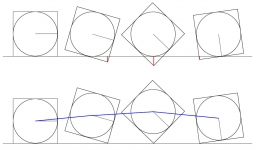Did you find an error or inaccuracy? Feel free to write us. Thank you!#### You need to know the following knowledge to solve this word math problem:

We encourage you to watch this tutorial video on this math problem:

## Related math problems and questions:

• Perimeter from areaWhat is the perimeter of the square if its content is 64 cm2?
• Fence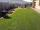The square garden has an area of 537 m2. How many meters is netting necessary to fence the garden?
• Two sides paintThe door has the shape of a rectangle with dimensions of 260cm and 170cm. How many cans of paint will be needed to paint this door if one can of paint cover 2m2 of the area? We paint the doors on both sides.
• Do you solve this?Determine area S of rectangle and length of its sides if its perimeter is 102 cm.
• Circles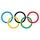For the circle c1(S1; r1=146 cm) and c2(S2; r2 = 144 cm) is distance of centers |S1S2| = 295 cm. Determine the distance between the circles.
• SquaresCalculate the area of square and result round to square decimeters. a) a = 1,52 dm b) a = 13 268mm c) a = 562 cm d) a = 1,52 m
• Rhombus sidesfind the sides of a rhombus, it area is 550 cm square and altitude is 55cm
• Height to the baseThe triangle area is 35 cm ^ 2. The size of the base is 10 cm. Find the length of height to the base.
• Two squaresTwo squares whose sides are in the ratio 5:2 have a sum of its perimeters 73 cm. Calculate the sum of the area of these two squares.
• Area of squareCalculate the content area of the square whose perimeter is 24 dm.
• Four prismsQuestion No. 1: The prism has the dimensions a = 2.5 cm, b = 100 mm, c = 12 cm. What is its volume? a) 3000 cm2 b) 300 cm2 c) 3000 cm3 d) 300 cm3 Question No.2: The prism base is a rhombus with a side length of 30 cm and a height of 27 cm. The height of tFind the radius of the circle with area S = 200 cm².The garden has the square shape with circumference 124 m. Divide it into two rectangular gardens, one should circumference 10 meters more than the second. What size will have a gardens?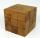Wood cube with edge 11 cm weights 0.753 kg. What weight have 10, 100 and 1000 these cubes?The cube of 5 cm chocolate roll weighs 30 g. How many calories will contain the same chocolate roller of a prism shape with a length of 0.5 m whose cross-section is an isosceles trapezoid with bases 25 and 13 cm and legs 10 cm? You know that 100 g of thisThe perimeter of a rectangle is 60cm. If the length of the rectangle is 20cm. a)find the width b)find the area.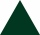The perimeter of an equilateral triangle is 33cm. How long is each side?